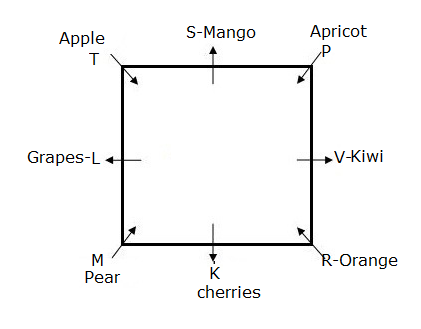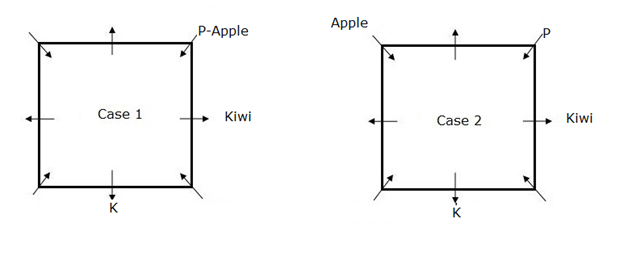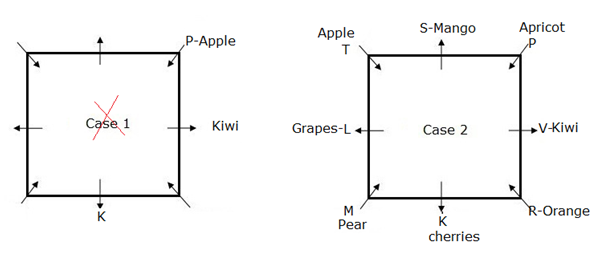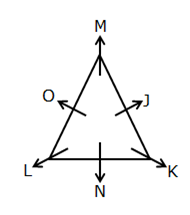# IBPS PO Prelims Reasoning (Day-15)

Dear Aspirants, Our IBPS Guide team is providing new series of Reasoning Questions for IBPS PO Prelims 2020 so the aspirants can practice it on a daily basis. These questions are framed by our skilled experts after understanding your needs thoroughly. Aspirants can practice these new series questions daily to familiarize with the exact exam pattern and make your preparation effective.

Start Quiz

Square seating

Directions (1-5): Study the following information carefully and answer the questions given below:

Eight persons L, M, K, R, P, S, T and V are sitting around a square table. Each one of them likes different fruits such as Apple, Mango, Grapes, Cherries, Pear, Kiwi, Orange and Apricot. Four persons sit each of the corners and four persons sit at the middle of the sides. Those who are sitting at the corner facing the centre and those who are sitting at the middle side of the table faces away from the centre.

The one who likes Kiwi sits second to the left of K. Two persons sit between K and the one who likes Apple. The one who likes Kiwi sits immediate left of P, who does not face away from the centre. The one who likes Apricot sits third to the right of L. T sits second to the right of the one who likes Apricot. T faces thecentre. K and T are not immediate neighbours.  S likes Mango. S sits third to the left of M. S and L are not immediate neighbours. R likes Orange. Two persons sit between V and the one who likes Pear. L does not like cherries.

1) V likes which of the following fruit?

A. Grapes

B. Cherries

C. Apricot

D. Kiwi

E. None of these

2) How many person/s sits between T and the one who likes cherries, when counted from right of T?

A. One

B. Two

C. Three

D. Four

E. None of these

3) Which of the following person likes Pear?

A.L

B.P

C.K

D.M

E. None of these

4) Which of the followingstatement is true?

A.P and R are immediate neighbours

B. As many persons sit between T and V is same as between L and S (Either from left or Right)

C. Three persons sit between P and the one who likes cherries (Either from left or Right)

D.L sits second to the right of the one who likes cherries

E. None is true

5) Four of the following five are alike in a certain way based on the above arrangement. Find which one does not belongs to the group?

A. S-Kiwi

B. V-cherries

C. T-Pear

D. M-Orange

E. L-Kiwi

Inequality

Direction (6-9): In the following questions, the relationship between different elements is shown in the statements. The statements are followed by conclusions. Find the conclusion which logically follows the given statements.

6) Statement:

G ≥ R = P < T ≤ Q; A < R < K = O; R ≤ U > B = J

Conclusions:

I). A > Q

II). R ≤ T

III). A < U

A. Either I or II follows

B. Only II and III follow

C. Only I and II follow

D. Only III follows

E. Only I and III follow

7) Statement:

R = O; P ≤ J ≤ N = Q = O < T; N = K ≥ S

Conclusions:

I). S ≤ R

II). P ≤ K

III). J = R

A. Only I follow

B. Only II follows

C. Only III follows

D. Only I and II follow

E. Only II and III follow

8) Statement:

A ≤ B = K; J = N ≤ P = T ≥ O; K > N =Q

Conclusion:

I). J = Q

II). A < N

III). J > O

A. Only III follow

B. Only I follow

C. Only I and II follow

D. Only I and III follow

E.Only II and III follow

9) Statement:

S ≤ T = M; I = G < L < N = K ≤ U; T < L =O

Conclusion:

I). O < U

II). I < K

III). M = I

A. Only II and III follow

B. Only III follows

C. Only I and II follow

D. All follow

E. None follows

Seating

10) Six persons viz. J, K, L, M, N and O are seated in a triangular table facing away from the center where three persons seated in the corners and three persons seated in the sides of the table, but not necessarily in the same order. L sits exactly opposite to J who sits to the immediate right of M. K sits to the immediate left of N and at one of the corners. Who among the following sits third to the right of K?

A. M

B. J

C. O

D. L

E. Cannot be determinedThe one who likes Kiwi sits second to the left of K. Two persons sit between K and the one who likes Apple. The one who likes Kiwi sits immediate left of P, who does not face away from the centre.The one who likes Apricot sits third to the right of L. T sits second to the right of the one who likes Apricot. T faces thecentre. K and T are not immediate neighbours.  S likes Mango. S sits third to the left of M. S and L are not immediate neighbours. R likes Orange. Two persons sit between V and the one who likes Pear. L does not like cherries.Case 1 will be dropped because the one who likes Apricot sits third to the right of L. T sits second to the right of the one who likes Apricot.

Directions (6-9):

A > Q  ->  A < R = P < T ≤ Q ->   False

R ≤ T  ->   R = P < T ->  False

A < U  ->  A < R ≤ U ->  True

S ≤ R -> S ≤ K=N = Q = O = R->  True

P ≤ K-> P ≤ J ≤ N= K-> True

J = R-> J ≤ N = Q = O = R-> False

J = Q ->  J = N = Q ->   True

A < N ->  A ≤ B = K> N ->   False

J > O ->  J = N ≤ P = T ≥ O ->  False

O < U  ->  O = L < N = K ≤ U ->   True

I < K  ->  I = G < L < N = K ->  True

M = I  ->  M < L > G = I  ->  False